Скачать презентацию Physics of Semiconductor Devices Lecture 3. Carriers

lecture_3.pptx

• Размер: 407.6 Кб
• Автор:
• Количество слайдов: 18

## Описание презентации Physics of Semiconductor Devices Lecture 3. Carriers по слайдам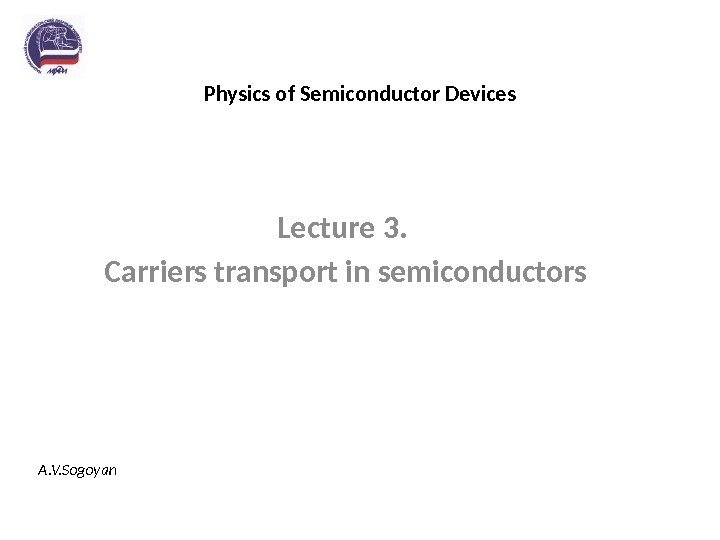Physics of Semiconductor Devices Lecture 3. Carriers transport in semiconductors A. V. SogoyanContents: • Carriers transport in semiconductors. Mobility. • Drift and diffusion currents.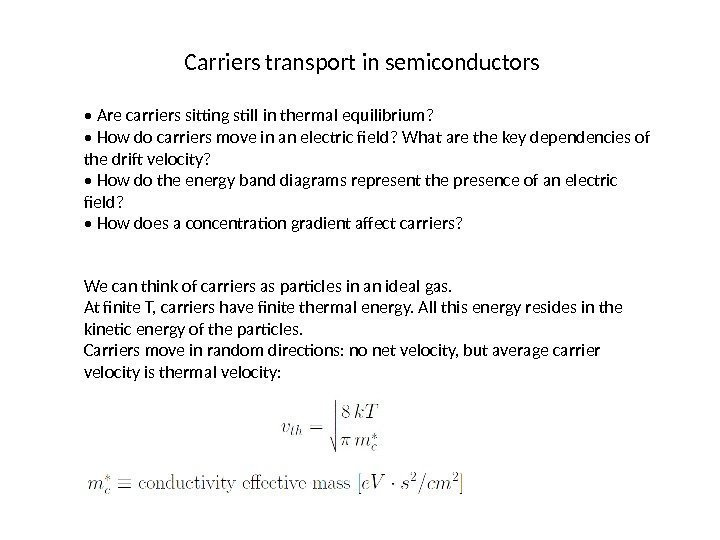Carriers transport in semiconductors • Are carriers sitting still in thermal equilibrium? • How do carriers move in an electric field? What are the key dependencies of the drift velocity? • How do the energy band diagrams represent the presence of an electric field? • How does a concentration gradient affect carriers? We can think of carriers as particles in an ideal gas. At finite T, carriers have finite thermal energy. All this energy resides in the kinetic energy of the particles. Carriers move in random directions: no net velocity, but average carrier velocity is thermal velocity: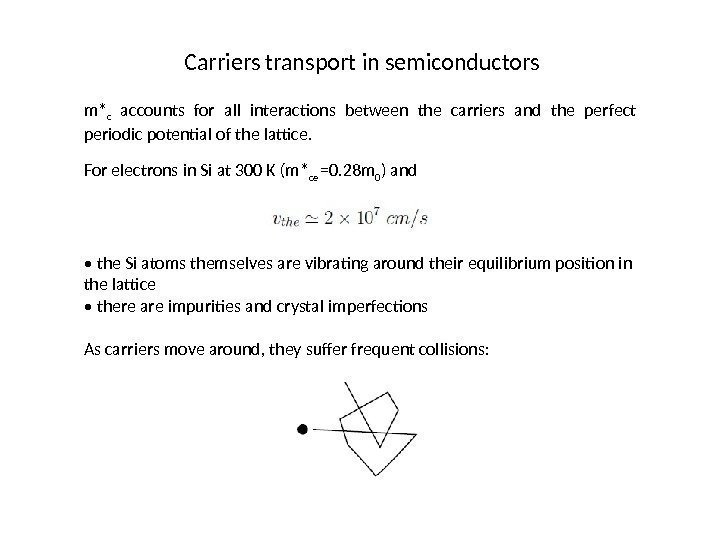Carriers transport in semiconductors m* c accounts for all interactions between the carriers and the perfect periodic potential of the lattice. For electrons in Si at 300 K (m* ce =0. 28 m 0 ) and • the Si atoms themselves are vibrating around their equilibrium position in the lattice • there are impurities and crystal imperfections As carriers move around, they suffer frequent collisions: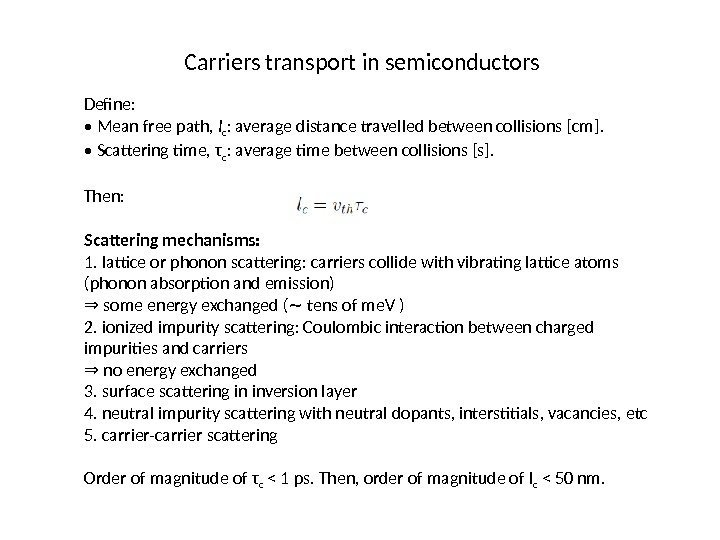Carriers transport in semiconductors Define: • Mean free path, l c : average distance travelled between collisions [cm]. • Scattering time, τ c : average time between collisions [s]. Then: Scattering mechanisms: 1. lattice or phonon scattering: carriers collide with vibrating lattice atoms (phonon absorption and emission) ⇒ some energy exchanged ( tens of me. V )∼ 2. ionized impurity scattering: Coulombic interaction between charged impurities and carriers ⇒ no energy exchanged 3. surface scattering in inversion layer 4. neutral impurity scattering with neutral dopants, interstitials, vacancies, etc 5. carrier-carrier scattering Order of magnitude of τ c < 1 ps. Then, order of magnitude of l c < 50 nm.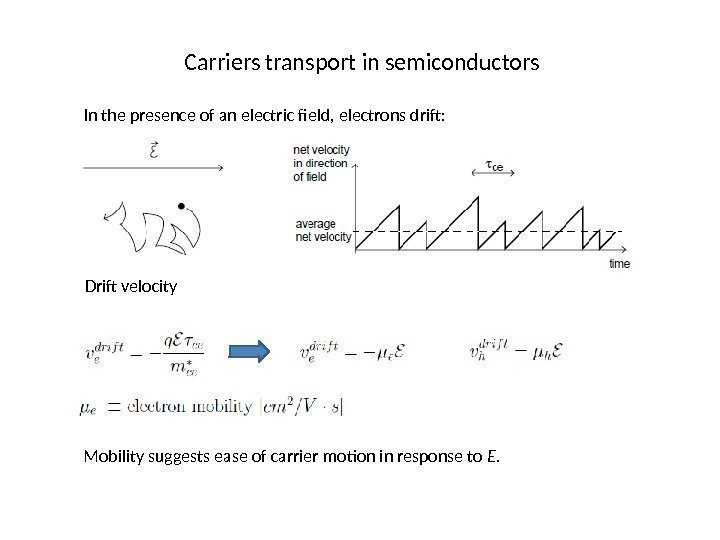Carriers transport in semiconductors In the presence of an electric field, electrons drift: Drift velocity Mobility suggests ease of carrier motion in response to E.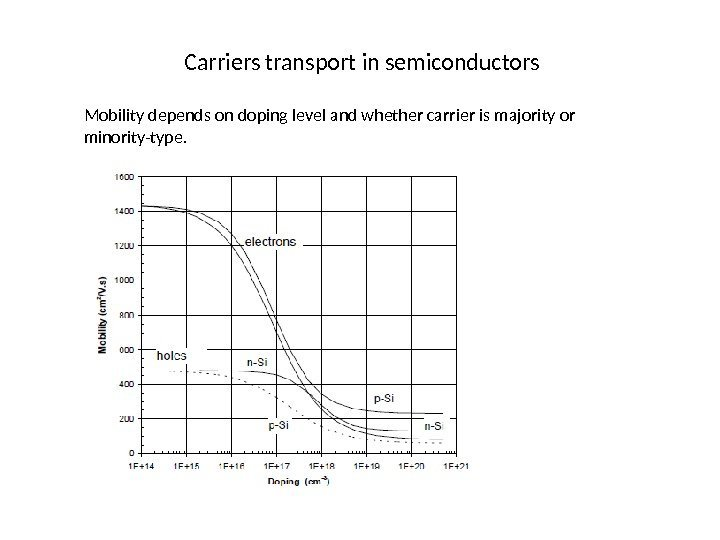Carriers transport in semiconductors Mobility depends on doping level and whether carrier is majority or minority-type.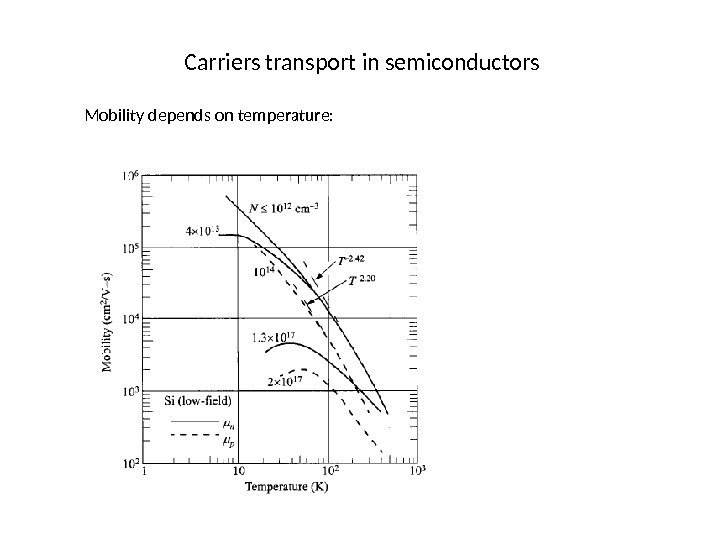Carriers transport in semiconductors Mobility depends on temperature: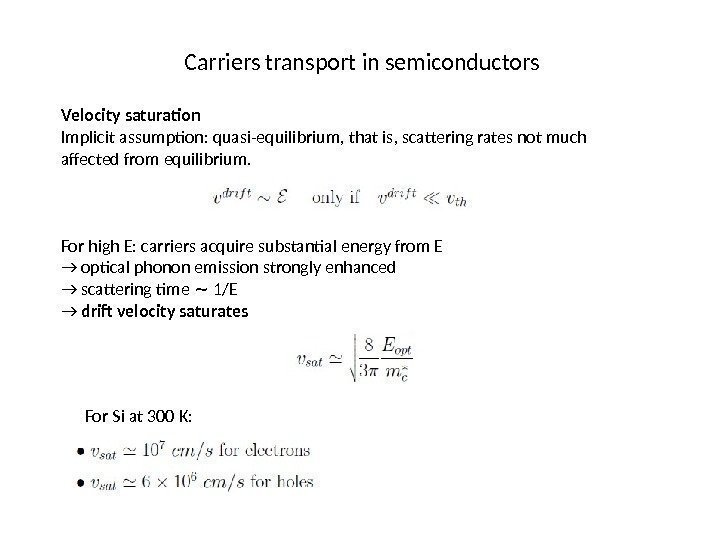Carriers transport in semiconductors Velocity saturation Implicit assumption: quasi-equilibrium, that is, scattering rates not much affected from equilibrium. For high E: carriers acquire substantial energy from E → optical phonon emission strongly enhanced → scattering time 1/E∼ → drift velocity saturates For Si at 300 K: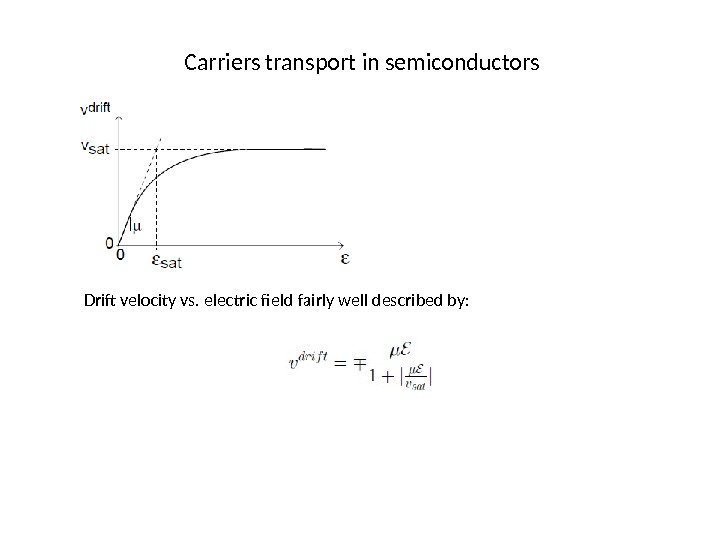Carriers transport in semiconductors Drift velocity vs. electric field fairly well described by: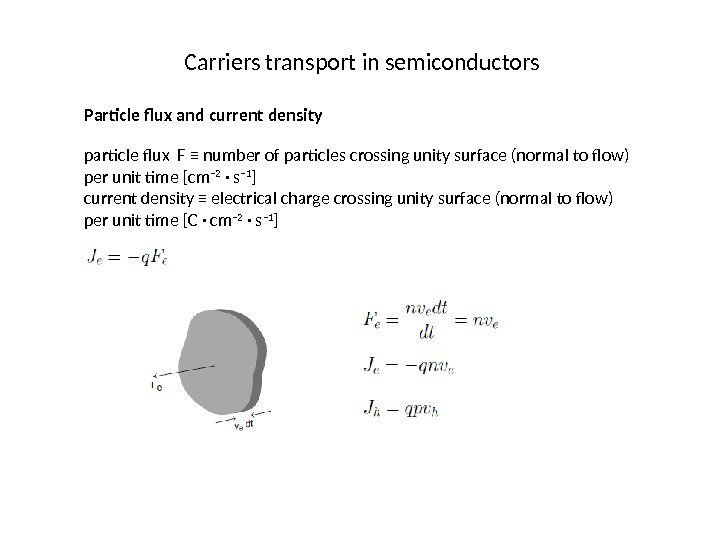Carriers transport in semiconductors Particle flux and current density particle flux F ≡ number of particles crossing unity surface (normal to flow) per unit time [cm − 2 · s − 1 ] current density ≡ electrical charge crossing unity surface (normal to flow) per unit time [C · cm − 2 · s − 1 ]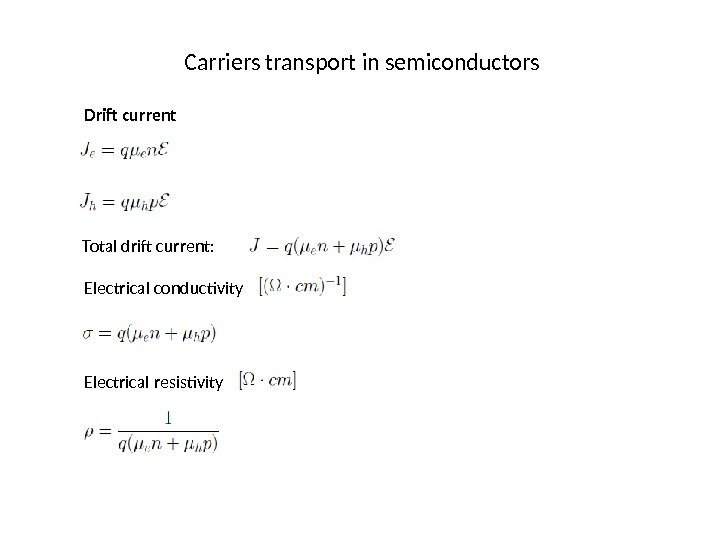Carriers transport in semiconductors Drift current Total drift current: Electrical conductivity Electrical resistivity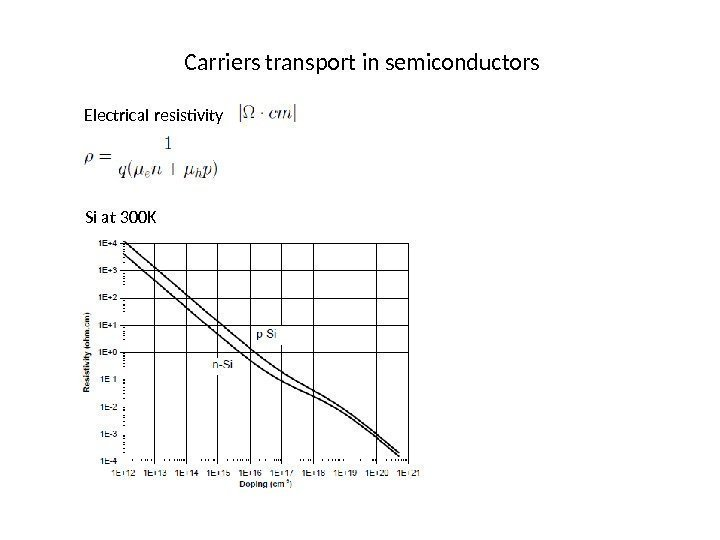Carriers transport in semiconductors Electrical resistivity Si at 300 K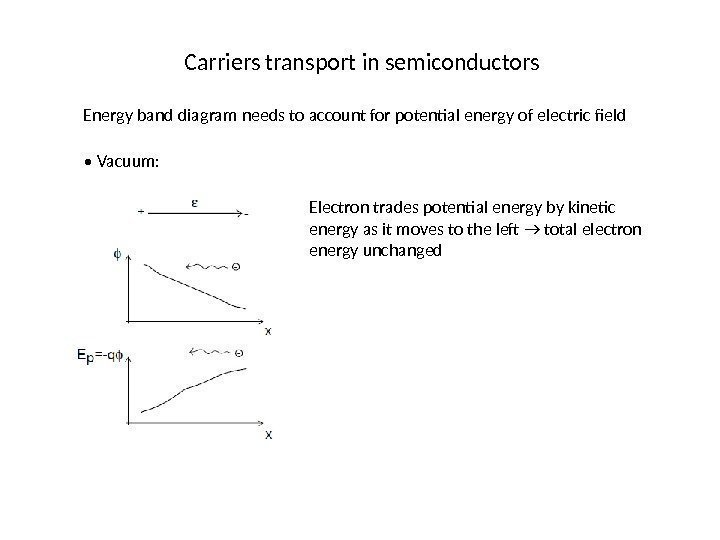Carriers transport in semiconductors Energy band diagram needs to account for potential energy of electric field • Vacuum: Electron trades potential energy by kinetic energy as it moves to the left → total electron energy unchanged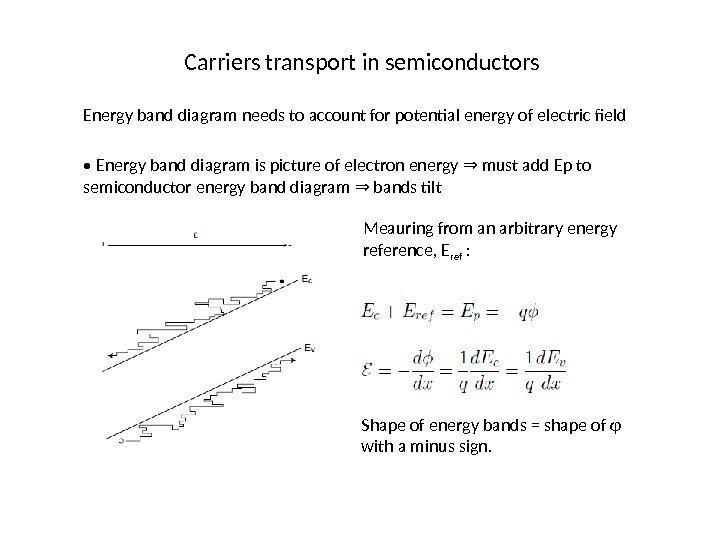Carriers transport in semiconductors Energy band diagram needs to account for potential energy of electric field • Energy band diagram is picture of electron energy must add Ep to ⇒ semiconductor energy band diagram bands tilt ⇒ Meauring from an arbitrary energy reference, E ref : Shape of energy bands = shape of φ with a minus sign.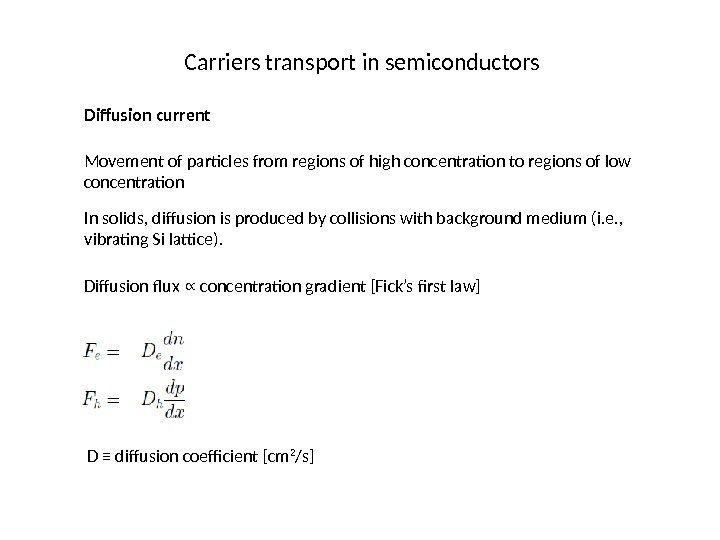Carriers transport in semiconductors Diffusion current Movement of particles from regions of high concentration to regions of low concentration In solids, diffusion is produced by collisions with background medium (i. e. , vibrating Si lattice). Diffusion flux concentration gradient [Fick’s first law]∝ D ≡ diffusion coefficient [cm 2 /s]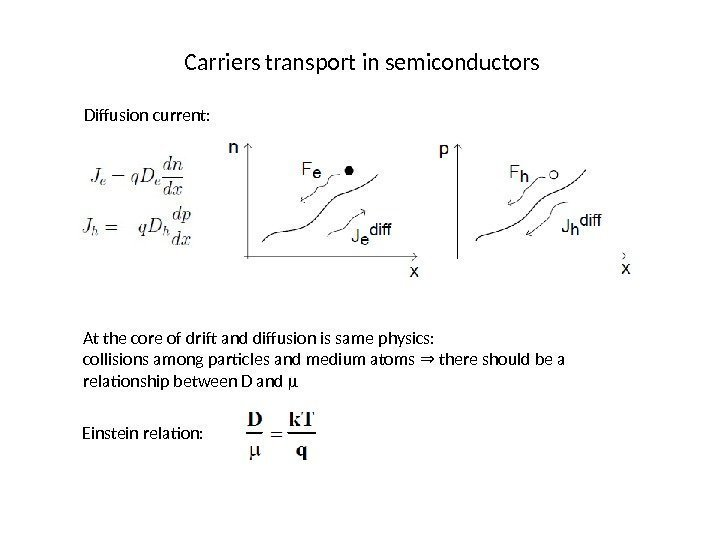Carriers transport in semiconductors Diffusion current: At the core of drift and diffusion is same physics: collisions among particles and medium atoms there should be a ⇒ relationship between D and µ Einstein relation: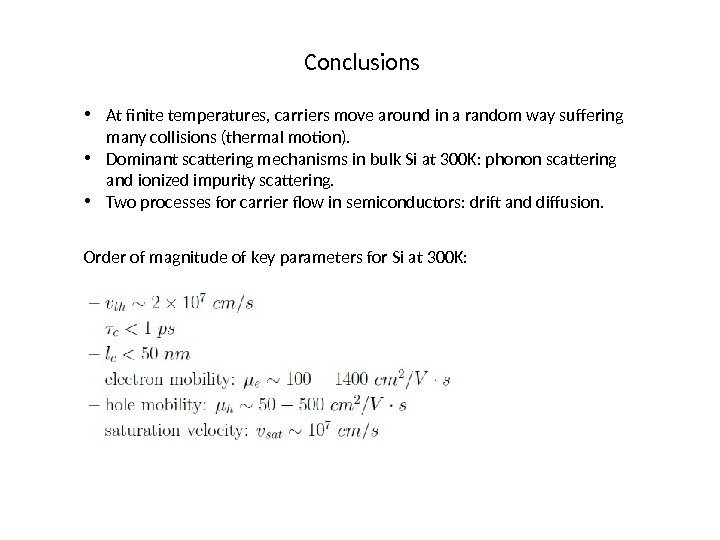Conclusions • At finite temperatures, carriers move around in a random way suffering many collisions (thermal motion). • Dominant scattering mechanisms in bulk Si at 300 K: phonon scattering and ionized impurity scattering. • Two processes for carrier flow in semiconductors: drift and diffusion. Order of magnitude of key parameters for Si at 300 K: